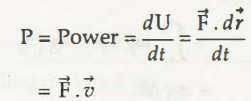Select Page

# Power

In general, power is defined as the rate of doing work. Coming to the mechanical power, it is the rate of energy that combines to bring certain output. In other words, power is the product of combination of number of forces and the velocity of the object in the same direction of applied force.

Mathematically, power can be written as-The S.I. unit of power is Joule/ second (J/s) which is termed as Watt. It is a scalar quantity and can also be written as,

1 Power = 1 N-m/ s

Links of Next Mechanical Engineering Topics:-

## Submit Assignment